# 1. 聚类简介

## 0x2：聚类的悖论

• ”相似对象归为一类，不相似对象归为不同类“这两个目标在很多情况下是互相冲突的。从数学上讲，虽然聚类共享具有等价关系甚至传递关系，但是相似性（或距离）不具有传递关系。具体而言，假定有一对象序列，X1，....，Xm，所有相邻元素（Xi-1、Xi+1）两两都非常相似，但是X1和Xm非常不相似。这种情况常常发生在cluster超过一定尺寸的时候，元素之间的传递性假设在这些场景下不一定100%成立。
• 聚类是一种无监督学习，即我们不能预测label，因此对于聚类，我们没有明确的成功评估过程。当然也不是完全没有任何的聚类效果评价指标，分类效用（category utility）就是一个理论上的评价指标。
• 对于一个给定的对象集合，可以有多种有意义的划分方式，这可能是因为对象间的距离（或相似性）有多种隐式的定义，例如将演讲者的录音根据演讲者的口音聚类或根据内容聚类。所以，给定一个数据集，有多种不同的聚类解决方案

## 0x3：聚类的公理化描述

### 1. 尺度不变性（SI）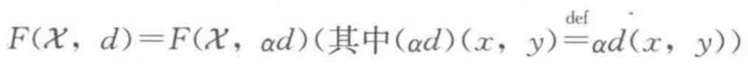### 2. 丰富性（Ri）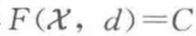### 3. 一致性（Co）

• 如果 x，y 属于同一类，则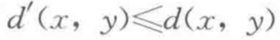• 如果 x，y 属于不同类，则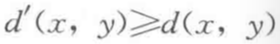## 0x5：聚类的大致分类

• 基于距离的聚类算法：基于距离的聚类算法是用各式各样的距离来衡量数据对象之间的相似度
• 基于密度的聚类方法：基于密度的聚类算法主要是依据合适的密度函数进行类间聚类
• 基于互连性的聚类算法：基于互连性的聚类算法通常基于图或超图模型，将高度连通的对象聚为一类

## 0x6：信息瓶颈 - 信息论视角下的聚类算法理解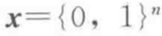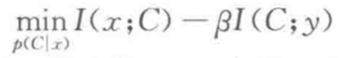• 一方面，我们希望文档属性和聚类属性的互信息尽可能小，这反映了我们希望对原始数据进行强压缩。
• 另一方面，我们希望聚类变量和词属性的互信息尽可能大，这反映了保留文档信息（用词在文档中出现来表示）的目标。将参数统计中的最小充分统计量推广到了任意分布。

## 0x7：分类效用（category utility） - 聚类效果评价指标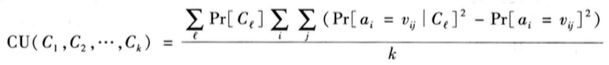• C1，C2，...，Ck 是 k 个聚类，外层的求和是针对每一个聚类的
• 内层两个求和分别是针对每个属性和每个实例的
• ai 代表第 i 个属性，它的值有 vi1，vi2，....，vij，一共要处理 j 个

• 聚类的意义不仅仅在于分类就完了，而更多是聚类后的结果对数据集的结构的发掘，对后续分类的帮助，即有利于更好地预测聚类中实例的属性值。
• 对于聚类结果 Cl 中的某个实例，属性 ai 的值为 vij 的概率是 Pr[ai=vij | Cl]，相对于概率 Pr[ai=vij]来说，是一个更好的估计，因为实例所在的聚类起到降低不确定性的作用，换句话说，聚类结果提供了有价值的信息，提高了分类结果的精确度。公式用两个概率的平方差来度量。
• 外部的 Pr[Cl] 以及除以 k 是考量整个聚类结果的复杂性，防止过度拟合。否与，由于概率是累计所有适当的实例获得的，每个实例单独作为一个聚类是数学上最好的结果。

《深入理解机器学习 - 从原理到算法》
http://blog.csdn.net/itplus/article/details/10087581
http://www.sohu.com/a/193777221_473283

# 2. K-Means算法(K-means clustering K均值聚类算法) - 基于硬划分的聚类

## 0x1：K-means算法模型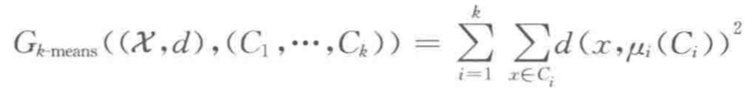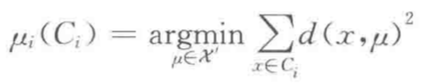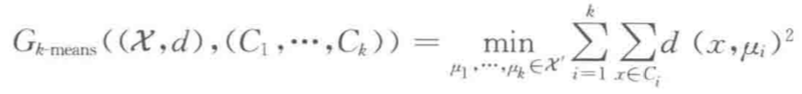k均值目标函数在实际的聚类应用中很常见。然而，事实证明寻找k均值（k-means）算法的最优解通常是计算不可行的（NP问题）。所以通常会用下面这种简单的迭代算法作为替代算法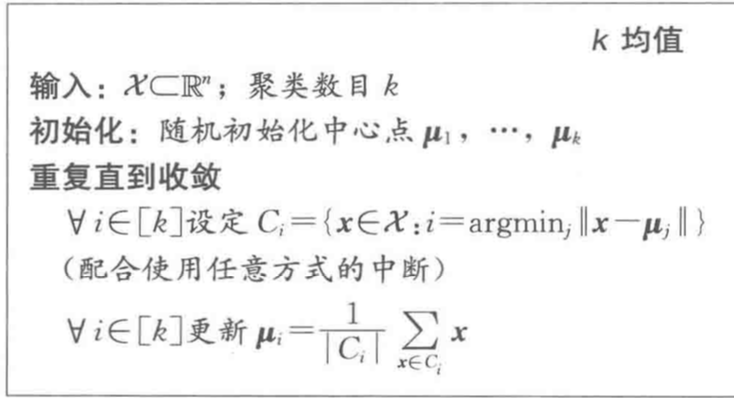• k均值算法的目标函数优化过程是单调非增的（也就是每次的迭代至少不会让结果更糟），但是 k均值算法本身对达到收敛的迭代次数并没有给出理论保证
• 算法给出的 k均值目标函数输出值和目标函数的最小可能值之差，并没有平凡下界，实际上，k均值可能会收敛到局部最小值。为了提高 k均值的结果，通常使用不同的随机初始化中心点，将该程序运行多次，并选取最优的结果。除此之外，有一些无监督的算法可以作为 k均值算法的前置算法，用来选取初始化中心。
• 在训练集上根据距离平方总和标准得到的”最佳“聚类，将不可避免地选择与数据点一样多的聚类！因为此时损失为0，为了抑制这种倾向，需要应用MDL准则进行模型结构复杂性惩罚，在模型复杂度和损失目标最优化之间寻求一个平衡。

## 0x2：K-means算法过程

kMeans聚类算法是数据挖掘十大算法之一，算法需要接受参数 k（k 个初始聚类中心）(也可由算法随机产生指定)，即将数据集进行聚类的数目和k个簇的初始聚类“中心”，结果是同一类簇中的对象相似度最高，不同类簇中的数据相似度最低，其聚类过程可以用下图表示### 2. 初始聚类中心(质心)的选择

Kmeans需要人为地确定初始聚类中心，不同的初始聚类中心可能导致完全不同的聚类结果。在实际使用中我们往往不知道我们的待聚类样本中哪些是我们关注的label，人工事先指定质心基本上是不现实的，在大多数情况下我们采取随机产生聚类质心这种策略

### 3. 确定了本轮迭代的质心后，将余下的样本点根据距离度量标准进行归类

#### p = 正无穷：距离等于各个坐标点的最大值### 4. 算法收敛（终止/停机）条件是什么？

• 这样不断进行"划分—更新—划分—更新"，直到每个簇的中心不在移动为止

## 0x3：Kmeans算法步骤图示描述

### 1. 步骤一 - 选取质心### 2. 步骤二 - 距离聚类### 3. 步骤三 - 重选新质心### 4. 步骤四（人生就是一个勇敢踏出第一步，然后不断自我否定和修正成长的过程）# -*- coding: utf-8 -*-

from sklearn.cluster import KMeans
import numpy as np

if __name__ == '__main__':
X = np.array([
[1, 2], [1, 4], [1, 0],
[4, 2], [4, 4], [4, 0]
])
kmeans = KMeans(n_clusters=2, random_state=0).fit(X)
print kmeans.labels_

kmeans.predict([[0, 0], [4, 4]])

print kmeans.cluster_centers_kMeans这种无监督聚类进行一次无监督聚类后，也可以得到一个分类器(classifier)，它是对当前train set的一个特征空间划分，基于这个分类器可以用于之后对新样本点的分类预测，这种就是有监督聚类

## 0x4：不同K值、不同的初始化中心点方式对Kmeans分类效果的影响

# -*- coding: utf-8 -*-

import numpy as np
import matplotlib.pyplot as plt
from mpl_toolkits.mplot3d import Axes3D

from sklearn.cluster import KMeans
from sklearn import datasets

if __name__ == '__main__':
np.random.seed(5)

centers = [[1, 1], [-1, -1], [1, -1]]
X = iris.data
y = iris.target

estimators = {'k_means_iris_3': KMeans(n_clusters=3),
'k_means_iris_8': KMeans(n_clusters=8),

fignum = 1
for name, est in estimators.items():
fig = plt.figure(fignum, figsize=(4, 3))
plt.clf()
ax = Axes3D(fig, rect=[0, 0, .95, 1], elev=48, azim=134)

plt.cla()
est.fit(X)
labels = est.labels_

ax.scatter(X[:, 3], X[:, 0], X[:, 2], c=labels.astype(np.float))

ax.w_xaxis.set_ticklabels([])
ax.w_yaxis.set_ticklabels([])
ax.w_zaxis.set_ticklabels([])
ax.set_xlabel('Petal width')
ax.set_ylabel('Sepal length')
ax.set_zlabel('Petal length')
fignum = fignum + 1

# Plot the ground truth
fig = plt.figure(fignum, figsize=(4, 3))
plt.clf()
ax = Axes3D(fig, rect=[0, 0, .95, 1], elev=48, azim=134)

plt.cla()

for name, label in [('Setosa', 0),
('Versicolour', 1),
('Virginica', 2)]:
ax.text3D(X[y == label, 3].mean(),
X[y == label, 0].mean() + 1.5,
X[y == label, 2].mean(), name,
horizontalalignment='center',
bbox=dict(alpha=.5, edgecolor='w', facecolor='w'))
# Reorder the labels to have colors matching the cluster results
y = np.choose(y, [1, 2, 0]).astype(np.float)
ax.scatter(X[:, 3], X[:, 0], X[:, 2], c=y)

ax.w_xaxis.set_ticklabels([])
ax.w_yaxis.set_ticklabels([])
ax.w_zaxis.set_ticklabels([])
ax.set_xlabel('Petal width')
ax.set_ylabel('Sepal length')
ax.set_zlabel('Petal length')
plt.show()## 0x5：K-means在图像处理上的应用

### 1. Color Quantization(真彩色降维低彩色) using K-Means

# -*- coding: utf-8 -*-

import numpy as np
import matplotlib.pyplot as plt
from sklearn.cluster import KMeans
from sklearn.metrics import pairwise_distances_argmin
from sklearn.utils import shuffle
from time import time

if __name__ == '__main__':
n_colors = 64

# Load the Summer Palace photo

# Convert to floats instead of the default 8 bits integer coding. Dividing by
# 255 is important so that plt.imshow behaves works well on float data (need to
# be in the range [0-1])
china = np.array(china, dtype=np.float64) / 255

# Load Image and transform to a 2D numpy array.
w, h, d = original_shape = tuple(china.shape)
assert d == 3
image_array = np.reshape(china, (w * h, d))

# 用1000张图像来训练出一个Kmeans分类器
print("Fitting model on a small sub-sample of the data")
t0 = time()
image_array_sample = shuffle(image_array, random_state=0)[:1000]
kmeans = KMeans(n_clusters=n_colors, random_state=0).fit(image_array_sample)
print("done in %0.3fs." % (time() - t0))

# 用训练好的Kmeans分类器对本例中的"夏宫"图像进行聚类处理，实际上就是在降维
# Get labels for all points
print("Predicting color indices on the full image (k-means)")
t0 = time()
labels = kmeans.predict(image_array)
print("done in %0.3fs." % (time() - t0))

codebook_random = shuffle(image_array, random_state=0)[:n_colors + 1]
print("Predicting color indices on the full image (random)")
t0 = time()
labels_random = pairwise_distances_argmin(codebook_random,
image_array,
axis=0)
print("done in %0.3fs." % (time() - t0))

def recreate_image(codebook, labels, w, h):
"""Recreate the (compressed) image from the code book & labels"""
d = codebook.shape
image = np.zeros((w, h, d))
label_idx = 0
for i in range(w):
for j in range(h):
image[i][j] = codebook[labels[label_idx]]
label_idx += 1
return image

# Display all results, alongside original image
plt.figure(1)
plt.clf()
ax = plt.axes([0, 0, 1, 1])
plt.axis('off')
plt.title('Original image (96,615 colors)')
plt.imshow(china)

plt.figure(2)
plt.clf()
ax = plt.axes([0, 0, 1, 1])
plt.axis('off')
plt.title('Quantized image (64 colors, K-Means)')
plt.imshow(recreate_image(kmeans.cluster_centers_, labels, w, h))

plt.figure(3)
plt.clf()
ax = plt.axes([0, 0, 1, 1])
plt.axis('off')
plt.title('Quantized image (64 colors, Random)')
plt.imshow(recreate_image(codebook_random, labels_random, w, h))
plt.show()## 0x6：从混合模型和EM算法的角度来谈kmeans聚类

### 1. 用形式化方式描述Kmeasn（公式9.1）

•的最优化（E期望）：首先，我们为选择一些初始值。然后在第一阶段，我们保持固定，关于最小化 J
•的最优化（M最大化）：在第二阶段，我们保持固定，关于最小化 J。不断重复这2个阶段优化直到收敛（1-of-k）http://blog.itpub.net/12199764/viewspace-1479320/
https://blog.yueyu.io/p/1614
http://scikit-learn.org/stable/modules/generated/sklearn.cluster.KMeans.html
http://scikit-learn.org/stable/auto_examples/cluster/plot_cluster_iris.html#sphx-glr-auto-examples-cluster-plot-cluster-iris-py
http://scikit-learn.org/stable/auto_examples/cluster/plot_color_quantization.html#sphx-glr-auto-examples-cluster-plot-color-quantization-py
http://scikit-learn.org/stable/auto_examples/cluster/plot_face_compress.html#sphx-glr-auto-examples-cluster-plot-face-compress-py
http://blog.csdn.net/gamer_gyt/article/details/51244850
http://scikit-learn.org/stable/auto_examples/cluster/plot_kmeans_assumptions.html#sphx-glr-auto-examples-cluster-plot-kmeans-assumptions-py
http://scikit-learn.org/stable/auto_examples/cluster/plot_kmeans_digits.html#sphx-glr-auto-examples-cluster-plot-kmeans-digits-py
http://chunqiu.blog.ustc.edu.cn/?p=435#comment-3556
http://www.cnblogs.com/bourneli/p/3645049.html
http://www.cnblogs.com/bourneli/p/3645049.html
https://en.wikipedia.org/wiki/Silhouette_(clustering)
https://kapilddatascience.wordpress.com/2015/11/10/using-silhouette-analysis-for-selecting-the-number-of-cluster-for-k-means-clustering/
http://scikit-learn.org/stable/auto_examples/cluster/plot_kmeans_silhouette_analysis.html#sphx-glr-auto-examples-cluster-plot-kmeans-silhouette-analysis-py
http://scikit-learn.org/stable/auto_examples/cluster/plot_kmeans_stability_low_dim_dense.html#sphx-glr-auto-examples-cluster-plot-kmeans-stability-low-dim-dense-py
http://scikit-learn.org/stable/modules/clustering.html#mini-batch-kmeans
http://scikit-learn.org/stable/auto_examples/cluster/plot_mini_batch_kmeans.html#sphx-glr-auto-examples-cluster-plot-mini-batch-kmeans-py
http://scikit-learn.org/stable/auto_examples/text/document_clustering.html#sphx-glr-auto-examples-text-document-clustering-pyhttp://coolshell.cn/articles/7779.htm

# 3. K-Means++算法

## 0x1：从爬山法问题中切入谈kmeans的缺陷以及kmeans++缓解了什么问题

• 从山上的某个随机位置出发开始
• Repeast
• 每次都都沿着“更高”的方向走一步
• Until直到某一步发现无论往任何方向走都不会更高k-means算法和上面的爬山法类似，不能保证最后能够找到最优的划分簇。这是因为算法一开始选择的是随机质心，基于随机质心，算法只能找到局部最优化分簇（例如图像的B点）。对于kmeans这种EM过程来说，最终的聚类结果严重依赖于初始中心点的选择

K-Means主要有两个最重大的缺陷 - 都和初始值有关

• K需要事先给定，这个K值的选定是非常难以估计的。很多时候，事先并不知道给定的数据集应该分成多少个类别才最合适
• K-Means算法需要用初始随机种子点来启动优化，这个随机种子点太重要，不同的随机种子点会有得到完全不同的结果

## 0x2：k-Means++算法步骤

• 从输入的数据点集合中随机选择一个点作为第一个种子点(聚类中心)
• 对于数据集中的每一个点x，计算它与最近聚类中心(指已选择的聚类中心)的距离D(x)并保存在一个数组里，然后把这些距离加起来得到Sum(D(x))
• 选择一个新的数据点作为新的聚类中心，选择的原则是: D(x)较大的点被选取作为聚类中心的概率较大(kMeans不同类别的距离越远越好)
• 先取一个能落在Sum(D(x))中的随机值Random
• 然后用Random -= D(x)，直到其结果 <= 0
• 选取在这个"递减过程"中D(x)最大的点，此时的点就是下一个"种子点"
• 重复2和3直到k个聚类中心被选出来
• 利用这k个初始的聚类中心来运行标准的k-means算法

#-*- coding:utf-8 -*-

import collections
import random
random.seed()

def roulette(datalist):
i = 0
soFar = datalist
ball = random.random()
while soFar < ball:
i += 1
soFar += datalist[i]
return datalist[i]

if __name__ == "__main__":
data = [
("dp1", 0.25),
("dp2", 0.4),
("dp3", 0.1),
("dp4", 0.15),
("dp5", 0.1)
]
results = collections.defaultdict(int)
for i in range(1000):
results[roulette(data)] += 1
print results## 0x3：Kmeans++算法策略

k-means++聚类的基本思想就是：虽然第一个中心点仍然随机选择，但其他的点则优先选择那些彼此相距很远的点

http://www.csdn.net/article/2012-07-03/2807073-k-means

# 4. 基于链接的层次聚类算法 - 基于分层的聚类（自底向上）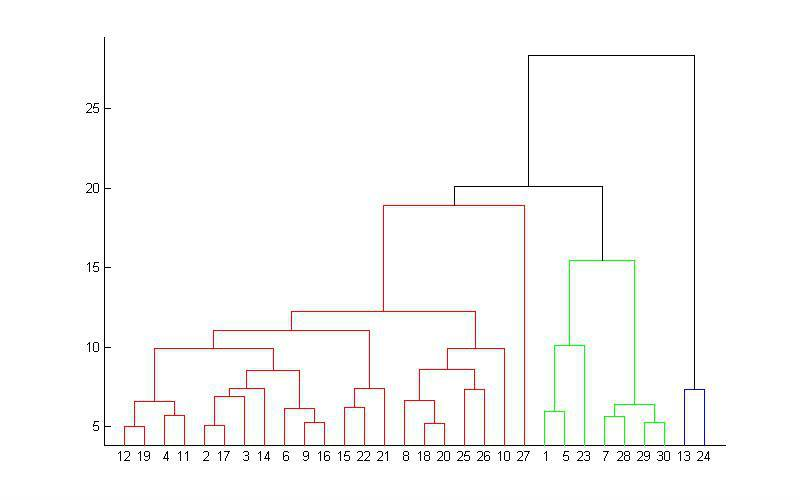• 聚类间距离度量：决定怎样测量（或定义）类间距离
• 提前终止条件：我们需要确定什么时候终止合并，否则最终只会得到一个单聚类

## 0x1：链接聚类模型

### 1. 聚类间距离度量公式

• 单链接最大链接聚类：类间距定义为两类元素间的最大距离（取两两组合最长距离的那个）。在这种度量标准下，单链接聚类会产生直径非常大的聚类。

• 完全链接聚类：只有两个聚类的所有成员都相似时，才认为两个聚类的距离很近、这种度量标准也对离群点很敏感，并且倾向于寻找紧凑的、直径较小的聚类。同时，也可能会出现一些实例与其他聚类的距离比与它自己所在聚类内其他点的距离还近很多的情况。

• 中心链接聚类：中心链接度量代表了聚类成员间最小和最大距离的一种折中，用聚类成员的中心来代表聚类。

#### 5）组平均（group-average）

• 组平均聚类：它使用合并后的聚类中的所有成员间的平均距离。这与平均聚类方法不同，它包含了相同原始聚类的平均值对。

### 2. 合并终止条件• 固定类的数量：固定参数 k，当聚类数目为 k 时停止聚类。使用这种停止准则需要我们对我们的场景要有较强的领域知识，即预先知道需要聚类的数量
• 设定距离上限：设定域子集间距最大上限，如果在某一轮迭代中，所有的组件距离都超过该阈值，则停止聚类

## 0x2：单链接/平均链接/最大链接聚类效果对比

# -*- coding:utf-8 -*-

from time import time

import numpy as np
from scipy import ndimage
from matplotlib import pyplot as plt

from sklearn import manifold, datasets

X = digits.data
y = digits.target
n_samples, n_features = X.shape

np.random.seed(0)

def nudge_images(X, y):
# Having a larger dataset shows more clearly the behavior of the
# methods, but we multiply the size of the dataset only by 2, as the
# cost of the hierarchical clustering methods are strongly
# super-linear in n_samples
shift = lambda x: ndimage.shift(x.reshape((8, 8)),
.3 * np.random.normal(size=2),
mode='constant',
).ravel()
X = np.concatenate([X, np.apply_along_axis(shift, 1, X)])
Y = np.concatenate([y, y], axis=0)
return X, Y

X, y = nudge_images(X, y)

#----------------------------------------------------------------------
# Visualize the clustering
def plot_clustering(X_red, X, labels, title=None):
x_min, x_max = np.min(X_red, axis=0), np.max(X_red, axis=0)
X_red = (X_red - x_min) / (x_max - x_min)

plt.figure(figsize=(6, 4))
for i in range(X_red.shape):
plt.text(X_red[i, 0], X_red[i, 1], str(y[i]),
color=plt.cm.spectral(labels[i] / 10.),
fontdict={'weight': 'bold', 'size': 9})

plt.xticks([])
plt.yticks([])
if title is not None:
plt.title(title, size=17)
plt.axis('off')
plt.tight_layout()

#----------------------------------------------------------------------
# 2D embedding of the digits dataset
print("Computing embedding")
X_red = manifold.SpectralEmbedding(n_components=2).fit_transform(X)
print("Done.")

from sklearn.cluster import AgglomerativeClustering

for linkage in ('ward', 'average', 'complete'):
t0 = time()
clustering.fit(X_red)
print("%s : %.2fs" % (linkage, time() - t0))

plt.show()## 0x3：链接算法模型

### 3. 最大链接聚类算法

http://scikit-learn.org/stable/auto_examples/cluster/plot_digits_linkage.html#sphx-glr-auto-examples-cluster-plot-digits-linkage-py
http://scikit-learn.org/stable/modules/clustering.html#hierarchical-clustering 

# 5. DBSCAN（Density-Based Spatial Clustering of Application with Noise） - 基于密度的聚类算法

## 0x1：DBSCAN模型

### 1. ∈邻域（∈ neighborhood），称为 x 的∈邻域。显然，### 2. 密度（density），称为 x 的密度。注意，这里的密度是一个整数值，且依赖于半径

### 3. 核心点（core point），若（核心点阈值 minimum numberof points required to form a cluster），则称 x 为 X 的核心点。记由 X 中所有核心点构成的集合为，并记表示由 X 中的所有非核心点构成的集合

### 4. 边界点（border point）满足。即 x 的邻域中存在核心点，则称 x 为 X 的边界点。记由 X 中所有边界点构成的集合为### 5. 噪音点（noise point），则称 x 为噪音点### 6. 直接密度可达（directly density-reachable），则称 y 是从 x 直接密度可达的

### 7. 密度可达（density-reachable），若它们满足直接密度可达的，，则称是从密度可达的

### 8. 密度相连（density-connected），若 y 和 z 均是从 x 密度可达的，则称 y 和 z 是密度相连的。显然，密度相连具有对称性

### 9. 类（cluster）

（1）Maximality：若，且 y 是从 x 密度可达的，则（2）Connectivity：若，则 x，y 是密度相连的

## 0x2：DBSCAN算法过程

DBSCAN算法的核心思想如下：从某个选定的核心点出发，不断向密度可达的区域扩张，从而得到一个包含核心点和边界点的最大化区域，区域中任意亮点密度相连## 0x3：hello world！DBSCAN

# -*- coding:utf-8 -*-

import numpy as np

from sklearn.cluster import DBSCAN
from sklearn import metrics
from sklearn.datasets.samples_generator import make_blobs
from sklearn.preprocessing import StandardScaler

# #############################################################################
# Generate sample data
centers = [[1, 1], [-1, -1], [1, -1]]
X, labels_true = make_blobs(n_samples=750, centers=centers, cluster_std=0.4,
random_state=0)

X = StandardScaler().fit_transform(X)

# #############################################################################
# Compute DBSCAN
db = DBSCAN(eps=0.3, min_samples=10).fit(X)
print db.labels_
labels = db.labels_

# Number of clusters in labels, ignoring noise if present.
n_clusters_ = len(set(labels)) - (1 if -1 in labels else 0)

print('Estimated number of clusters: %d' % n_clusters_)
print("Homogeneity: %0.3f" % metrics.homogeneity_score(labels_true, labels))
print("Completeness: %0.3f" % metrics.completeness_score(labels_true, labels))
print("V-measure: %0.3f" % metrics.v_measure_score(labels_true, labels))
print("Silhouette Coefficient: %0.3f"
% metrics.silhouette_score(X, labels))

# #############################################################################
# Plot result
import matplotlib.pyplot as plt

# Black removed and is used for noise instead.
unique_labels = set(labels)
colors = [plt.cm.Spectral(each)
for each in np.linspace(0, 1, len(unique_labels))]
for k, col in zip(unique_labels, colors):
if k == -1:
# Black used for noise.
col = [0, 0, 0, 1]

plt.plot(xy[:, 0], xy[:, 1], 'o', markerfacecolor=tuple(col),
markeredgecolor='k', markersize=14)

plt.plot(xy[:, 0], xy[:, 1], 'o', markerfacecolor=tuple(col),
markeredgecolor='k', markersize=6)

plt.title('Estimated number of clusters: %d' % n_clusters_)
plt.show()http://shiyanjun.cn/archives/1288.html
https://en.wikipedia.org/wiki/DBSCAN
https://www.cnblogs.com/hdu-2010/p/4621258.html
http://blog.csdn.net/itplus/article/details/10088625
https://www.cnblogs.com/pinard/p/6208966.html
http://blog.csdn.net/xieruopeng/article/details/53675906
http://www.cnblogs.com/aijianiula/p/4339960.html
http://scikit-learn.org/stable/modules/generated/sklearn.cluster.DBSCAN.html

# 6. Clustering by fast search and find of density peaks（CFSFDP聚类）- 基于密度（Density）的聚类

## 0x1：算法思想

1. 聚类中心本身密度大，即它被密度均不超过它的邻居包围，或者说聚类中心是整个簇中密度最大的点；
2. 聚类中心与其他密度更大的数据点之间的“距离”相对更大；

## 0x2：算法模型

### 1. 局部密度ρi

#### 1）Cut-off kernel：阶跃统计函数，只关注数据点是否在dc阈值范围内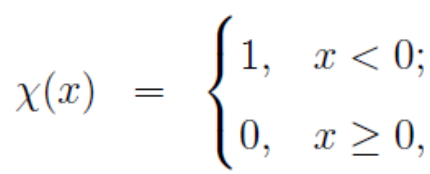#### 2）Gaussian kerne：柔性距离统计函数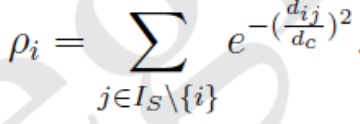### 2. 到高局部密度点的距离δi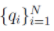表示数据点局部密度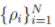的一个降序排列下标序，即它满足：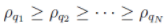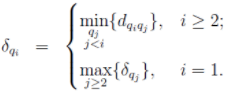1）当 Xi 具有最大局部密度时，δi 表示 S 中与 Xi 距离最大的数据点与 Xi 之间的距离；
2）否则，δi 表示在所有局部密度大于 Xi 的数据点中（即其他cluster centers），与 Xi 距离最小的那个（或那些）数据点与 Xi 之间的距离。

## 0x3：聚类过程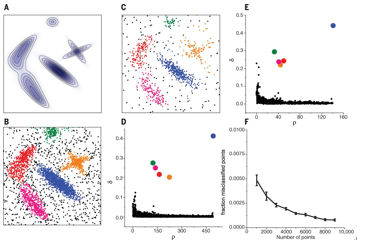## 0x4：算法形式化描述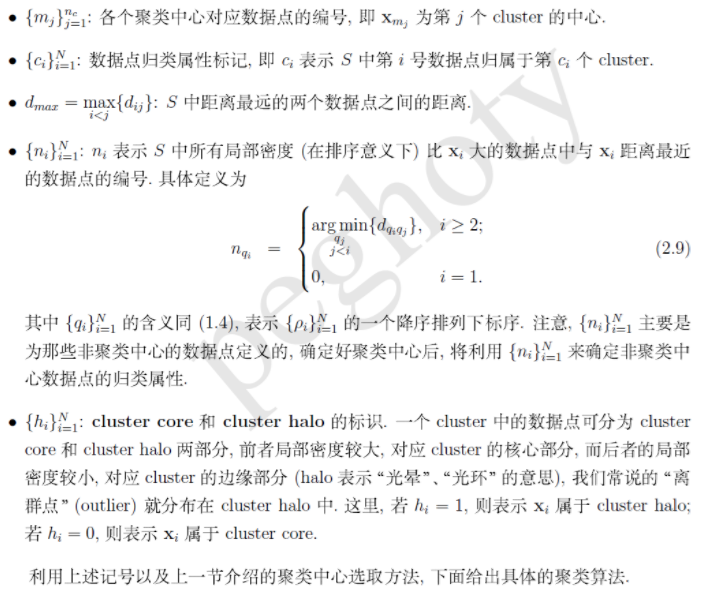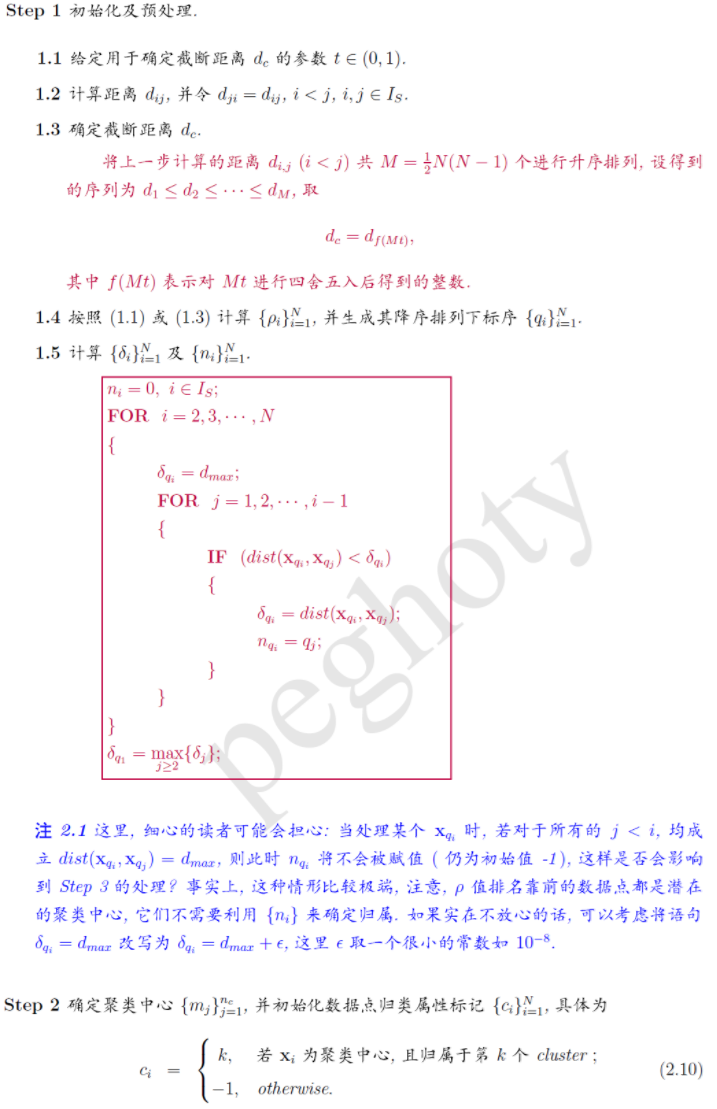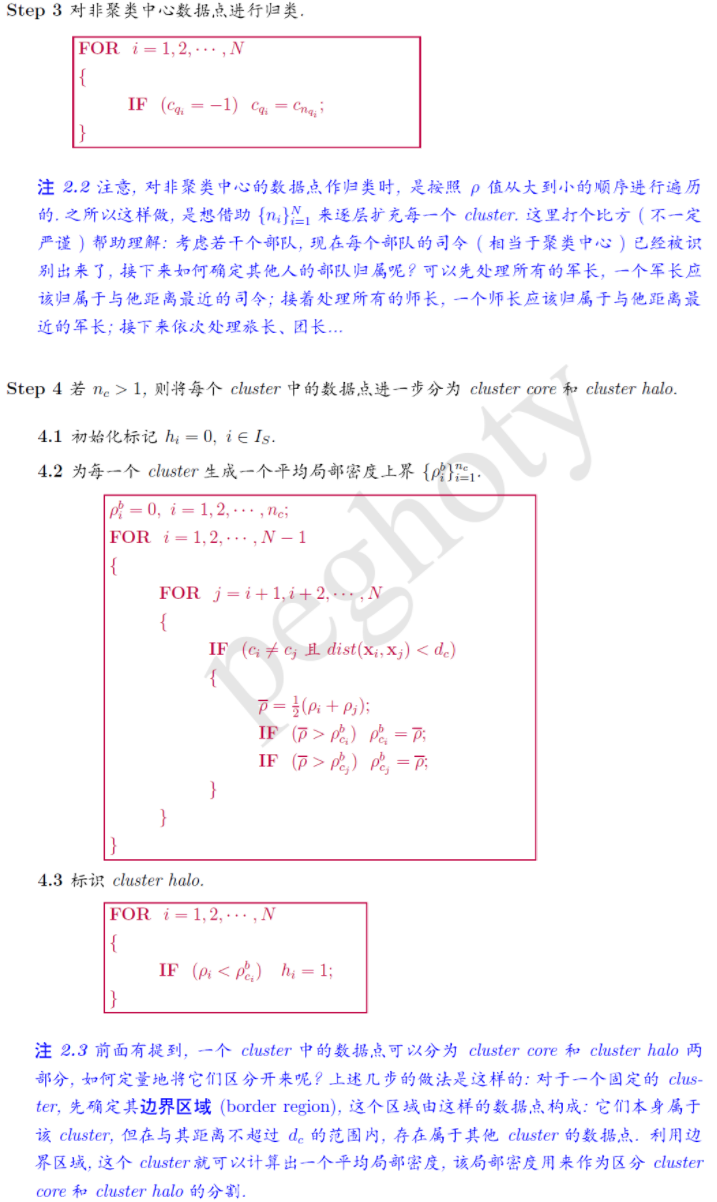http://science.sciencemag.org/content/344/6191/1492
https://segmentfault.com/a/1190000011337432
https://blog.csdn.net/itplus/article/details/38926837

# 7. SOM（Self-organizing Maps）- 基于模型的聚类（model-based methods）

目标数据集是由一系列的概率分布所决定的

http://www.cnblogs.com/LittleHann/p/7101992.html

# 8. GMM（Gaussian Mixture Model, 高斯混合模型）- 基于概率的聚类（probability-based methods）

## 0x1：传统硬分类（hard decision）聚类算法存在的挑战

• 以分类效用公式为驱动力的聚类算法，为了避免过度拟合必须选择除数k，即需要提供一个人为的聚类标准差最小值，以及为避免每个实例成为一个聚类的特定的截止值
• 增量启发式算法本身存在不确定性：
• 结果在多大程度上依赖于实例的输入顺序
• 合并、分裂等局部重建操作是否足以扭转由于不好的实例次序所带来的糟糕的初始决定
• 最终结构是否代表分类效用的局部最大值
• 无法知道最终的结果离全局最大值到底有多远
• 结果的层次性也不能回避哪个是最好的聚类这个问题
• 互斥的聚类划分引入了脆弱性，位于灰色地带的样本很可能会被错误地分到错误的聚类中。同时不小心引入的离群点可能会极大地破坏原本有效的聚类划分

## 0x2：概率聚类基本概念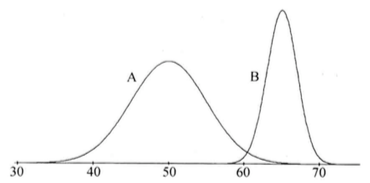• 聚类A的均值和标准差分别是μA和σA
• 聚类B的均值和标准差分别是μB和σB
• 从这些分布中抽样，聚类A的抽样概率为pA，聚类B的抽样概率为pB，pA+pB=1

## 0x4：混合模型预测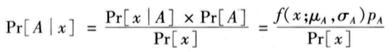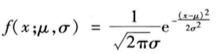https://baike.baidu.com/item/GMM/4258031?fr=aladdin